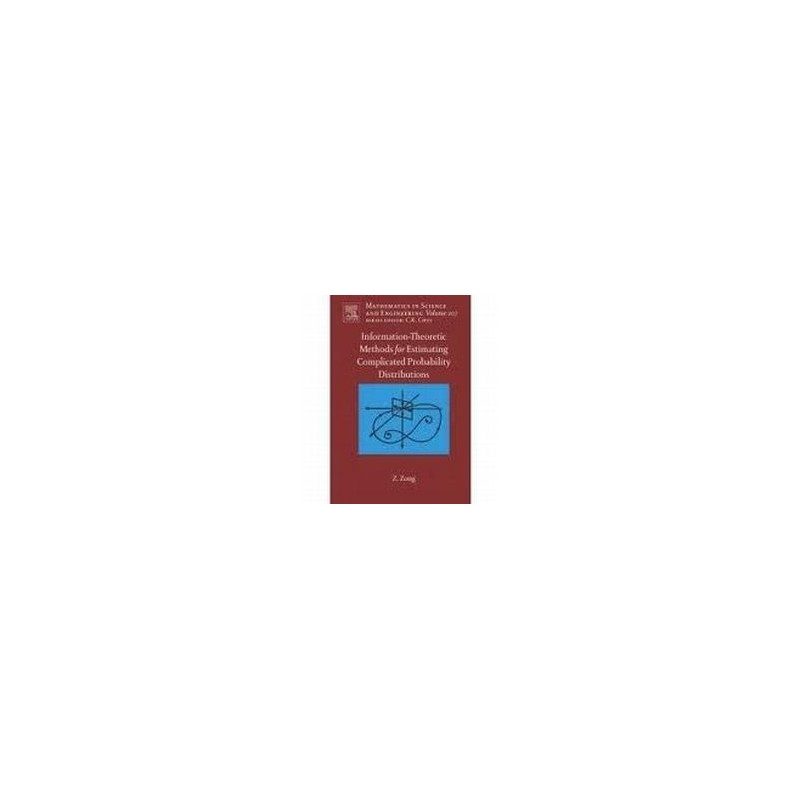﻿
Obniżka!Zobacz większe

# Traveling Wave Analysis of Partial Differential Equations

Graham Griffiths, William Schiesser

ID: 176984

### Więcej informacji

Although the Partial Differential Equations (PDE) models that are now studied are usually beyond traditional mathematical analysis, the numerical methods that are being developed and used require testing and validation. This is often done with PDEs that have known, exact, analytical solutions. The development of analytical solutions is also an active area of research, with many advances being reported recently, particularly traveling wave solutions for nonlinear evolutionary PDEs. Thus, the current development of analytical solutions directly supports the development of numerical methods by providing a spectrum of test problems that can be used to evaluate numerical methods.

This book surveys some of these new developments in analytical and numerical methods, and relates the two through a series of PDE examples. The PDEs that have been selected are largely "named'' since they carry the names of their original contributors. These names usually signify that the PDEs are widely recognized and used in many application areas. The authors' intention is to provide a set of numerical and analytical methods based on the concept of a traveling wave, with a central feature of conversion of the PDEs to ODEs.

• Includes a spectrum of applications in science, engineering, applied mathematics

• Presents a combination of numerical and analytical methods

• Provides transportable computer codes in Matlab and Maple

1. Traveling wave, residual function methods for analytical solutions to PDEs;
3. Linear diusion equation;
4. Linear convection diusion reaction equation;
5. Diusion equation with nonlinear source terms;
6. Burgers-Huxley equation;
7. Burgers-Fisher equation;
8. Fisher-Kolmogorov equation;
9. Fitzhugh-Nagumo equation;
10. Fisher-Kolmogorov-Petrovskii-Piskunov equation;
11. Kuramoto-Sivashinsky equation;
12. Kawahara equation;
13. Benjamin-Bona-Mahoney (RLW) equation;
14. Extended Bernoulli equation;
15. Hyperbolic Liouville equation;
16. Sine-Gordon equation;
17. Mth order Klein-Gordon equation;
18. Boussinesq equation;
19. Modied wave equation;
20. Appendix 1 - Analytical solution methods for traveling wave problems;